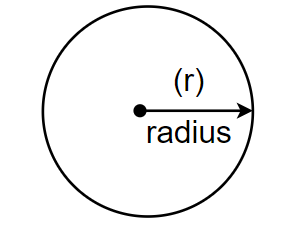QuestionAnswers

# Recall, $\pi$ is defined as the ratio of the circumference (say c) of a circle to its diameter (say d). That is, $\pi =\dfrac{c}{d}$. This seems to contradict the fact that $\pi$ is irrational. How will you resolve this contradiction?

Hint: First, we can try to recollect the value of $\pi$ to as many digits as you can. Now think of a scale or any other measuring device that gives you an exact value of a quantity or its approximate value. Use these claims to resolve the contradiction.
We have been given $\pi =\dfrac{c}{d}$, that is the ratio of the circumference (c) of a circle to its diameter (d), but $\pi$ is irrational, so these two things seems to contradict each other. We have to resolve this contraction. Let us draw a circle first.Here the circumference of the circle (c) of the circle is given by $2\pi r$, where $r$ is the radius of the circle. So, we get, $c=2\pi r\ldots \ldots \ldots \left( i \right)$.
We also know that the diameter is twice the radius of a circle, so we get, $d=2r\ldots \ldots \ldots \left( ii \right)$. By dividing equation (i) and equation (ii), we get, $\dfrac{c}{d}=\dfrac{2\pi r}{2r}\Rightarrow \dfrac{c}{d}=\pi$.
Now when we measure a length with a scale or any other device, we may get an approximate value of that quantity. Therefore, we may not realise that circumference (c) or diameter (d) is irrational and hence their ratio is also irrational. Also, the value of $\pi$ is approximated as 3.14 or $\dfrac{22}{7}$, which is not exact because $\pi$ is irrational, so it would be non-terminating and non-repeating numbers. Hence we know now that $\dfrac{c}{d}$ is also irrational because we cannot find its exact value and the division of irrational numbers is also irrational.
Hence, we have resolved the contradiction of the value of $\pi$ as $\dfrac{c}{d}$.
Note: Here students must note that 3.14 is not the exact value of $\pi$, but only an approximation. The digits of $\pi$ are infinite like 3.141592653897932…… and so on because every irrational number is non-terminating and non-repeating. Also, a scale or any either device only gives us a value up to its least count and not the exact value. So, we cannot say the value of circumference and diameter that we measure are rational numbers. The diameter of a circle is always irrational as it contains $\pi$, in its circumference, that is $2\pi r$.